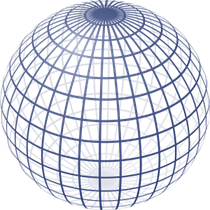# Surface Area of a Sphere

The surface area of a sphere is defined as the region covered by its outer surface in three-dimensional space.  A Sphere is a three-dimensional solid having a round shape, just like a circle. The formula of total surface area of a sphere in terms of pi (π) is given by:

 Surface area =  4 π r2 square units

The difference between a sphere and a circle is that a circle is a two-dimensional figure or a flat shape, whereas, a sphere is a three-dimensional shape. Therefore, the area of circle is different from  area of sphere.

Area of circle = π r

## Definition

From a visual perspective, a sphere has a three-dimensional structure that forms by rotating a disc that is circular with one of the diagonals.

Let us consider an instance where spherical ball faces are painted. To paint the whole surface, the paint quantity required has to be known beforehand. Hence, the area of every face has to be known to calculate the paint quantity for painting the same. We define this term as the total surface area.

The surface area of a sphere is equal to the areas of the entire face surrounding it.## Formula

The surface area of a sphere formula is given  by,

A = 4 π rsquare units

For any three-dimensional shapes, the area of the object can be categorised into three types. They are:

• Curved Surface Area
• Lateral Surface Area
• Total Surface Area

Curved Surface Area: The curved surface area is the area of all the curved regions of the solid.

Lateral Surface Area: The lateral surface area is the area of all the regions except bases (i.e., top and bottom).

Total Surface Area: The total surface area is the area of all the sides, top and bottom the solid object.

In case of a Sphere, it has no flat surface.

Therefore, the Total surface area of a sphere = Curved surface area of a sphere

## Solved Examples

 Example 1– Calculate the cost required to paint a football which is in the shape of a sphere having a radius of 7 cm. If the painting cost of football is INR 2.5/square cm. (Take π = 22/7) Solution  We know, The total surface area of a sphere =  4 π r2 square units = 4 × (22/7) × 7 × 7 = 616 cm2 Therefore, total cost of painting the container = 2.5 × 616 = Rs. 1540 Example 2- Calculate the curved surface area of a sphere having radius equals to 3.5 cm(Take π= 22/7) Solution– We know, Curved surface area = Total surface area = 4 π r2 square units = 4 × (22/7) × 3.5 × 3.5 Therefore, the curved surface area of a sphere= 154 cm2

To learn all concepts in Maths in a more engaging way, register at BYJU’S. Also, watch interesting videos on various maths topics by downloading BYJU’S– The Learning App.

## Frequently Asked Questions – FAQs

Q1

### What is the surface area of sphere?

The surface area of a sphere is total area covered by its outer surface in three dimensional space.
Q2

### How to find the surface area of a sphere?

To find the surface area of a sphere, we can use the formula:
SA = 4 π r2 square units
Where r is the radius of sphere
Q3

### What is the surface area of hemisphere?

Surface area of hemisphere is equal to the sum of its curved surface area and base area. The formula is given by: 3 π r2 square units
Q4

### What is the surface area of a cone?

The surface area of cone is the sum of its curved surface area and circular base area. The formula is given by:
TSA = πr(l+r)
Where l is the slant heigh of the cone and r is the radius of the circular base.
Q5

### What is lateral surface area of sphere?

Lateral surface area of sphere is nothing but its curved surface area.
Test your knowledge on Surface Area Of A Sphere